## 二、范数与正则项

• 非负性：$||\vec{x}||≥0$
• 齐次性：$||c·\vec{x}||=|c|||\vec{x}||$
• 三角不等式：$||\vec{x}+\vec{y}||≤||\vec{x}||+||\vec{y}||$

• $L_1-$范数：$||x⃗ ||=∑^d_{i=1}| x_i|$;
• $L_2-$范数：$||x⃗ ||_2=(∑^d_{i=1}x^2_i)^{1/2}$；
• $L_p-$范数：$||x||_p=(\sum_{i=1}^n|x_i|^p)^{\frac{1}{p}}$
• $L_∞-$范数：$||x⃗ ||_∞=lim_{p→+∞}(∑^d_{i=1}x^p_i)^{1/p}$。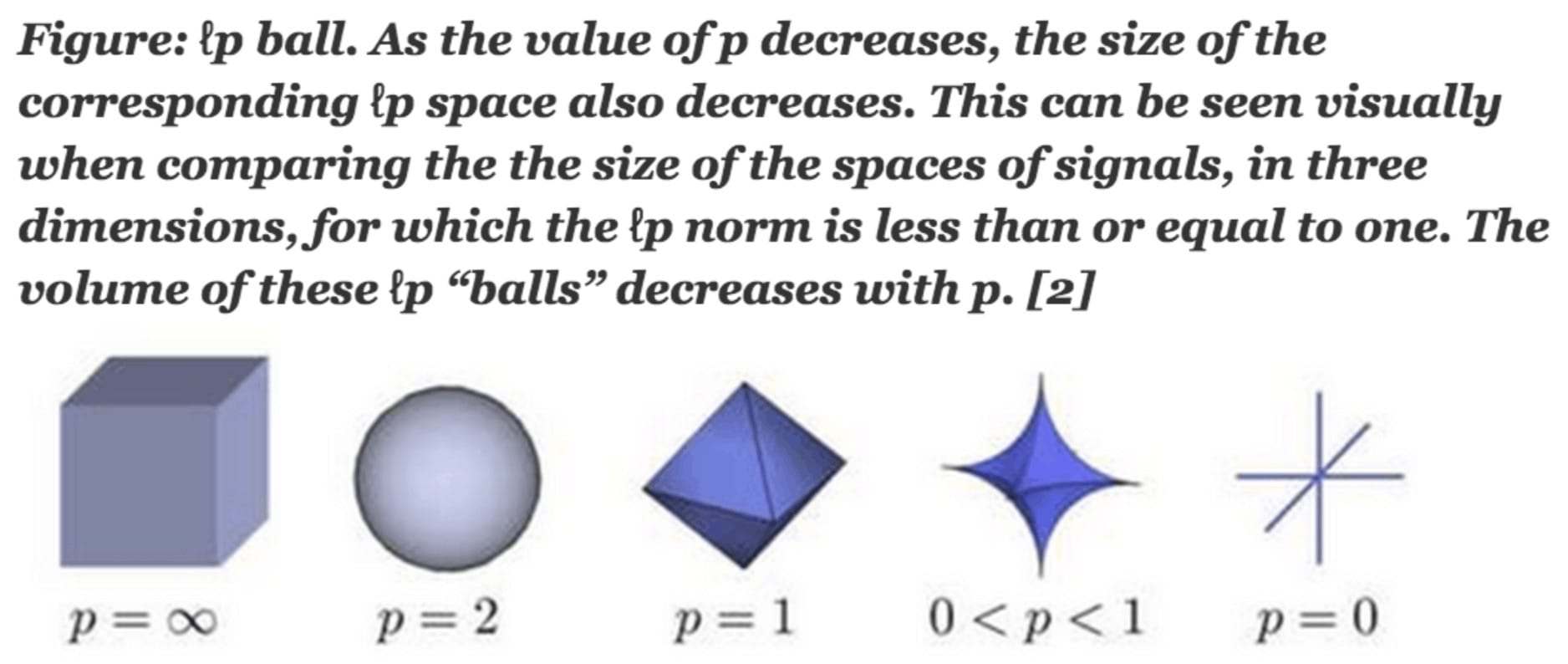• 非连续
• 非凸
• 不可求导

### 2.3 $L_1-$正则项与$L_2-$正则项的区别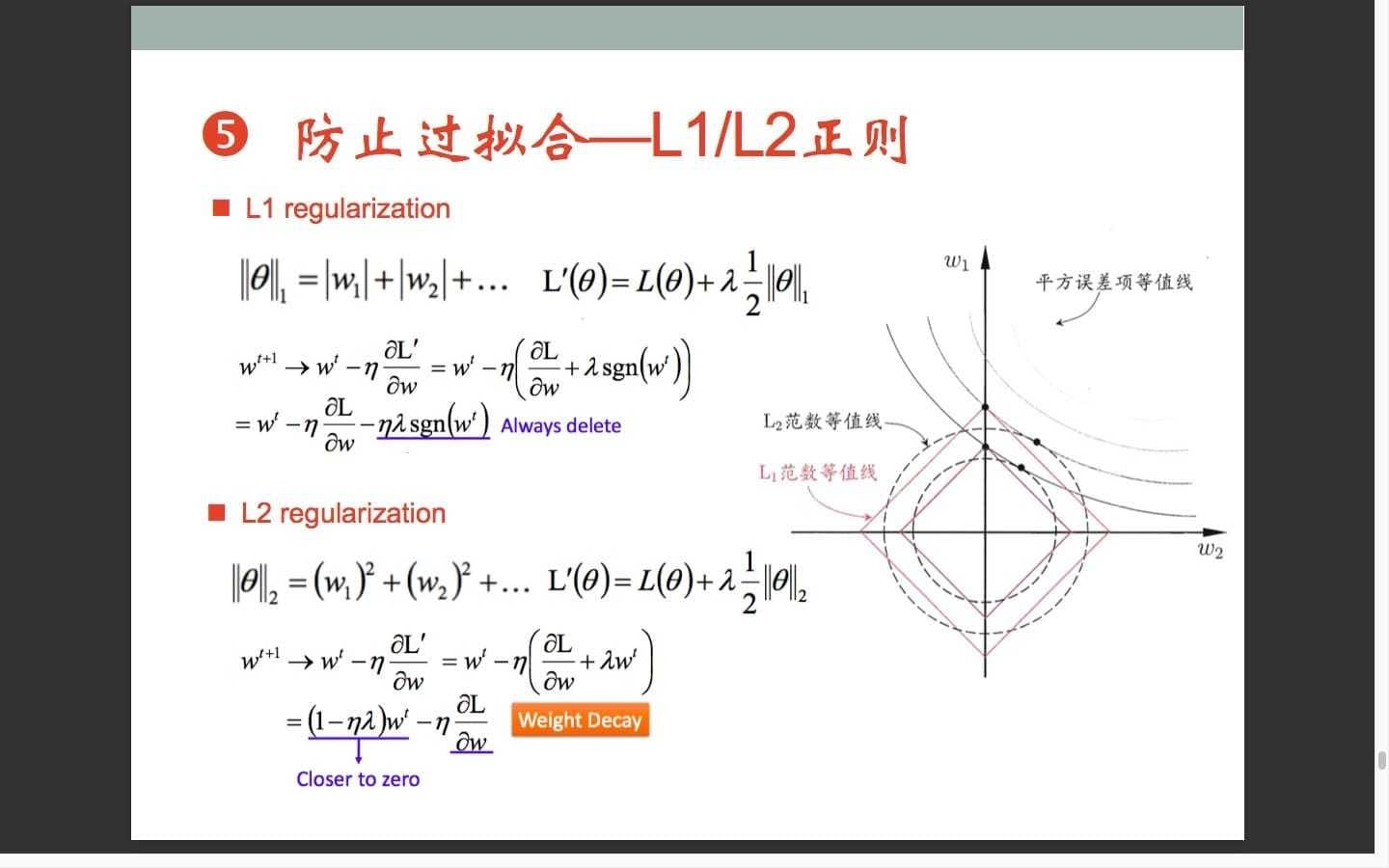## 三、贝叶斯先验

### 3.1 Linear Regression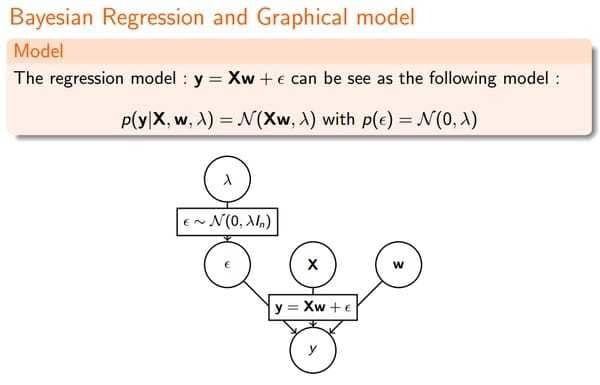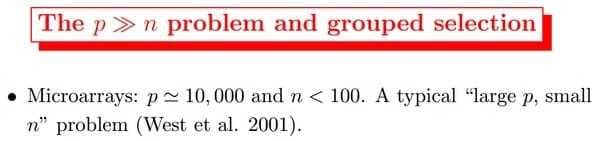### 3.2 Ridge Regression

Ridge Regression的提出就是为了解决multicolinearity的，加一个L2 penalty term也是因为算起来方便。然而它并不能shrink parameters to 0.所以没法做variable selection。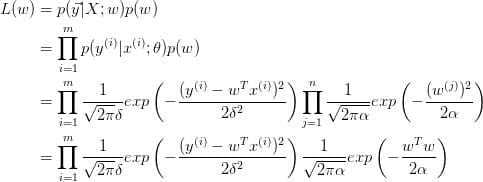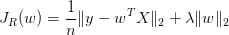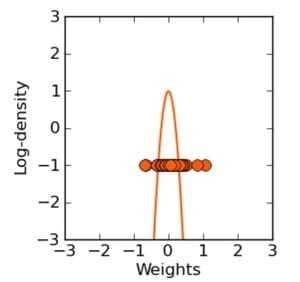Typically ridge or ℓ2 penalties are much better for minimizing prediction error rather than ℓ1 penalties. The reason for this is that when two predictors are highly correlated, ℓ1 regularizer will simply pick one of the two predictors. In contrast, the ℓ2 regularizer will keep both of them and jointly shrink the corresponding coefficients a little bit. Thus, while the ℓ1 penalty can certainly reduce overfitting, you may also experience a loss in predictive power.

### 3.3 LASSO

LASSO是针对Ridge Regression的没法做variable selection的问题提出来的，L1 penalty虽然算起来麻烦，没有解析解，但是可以把某些系数shrink到0。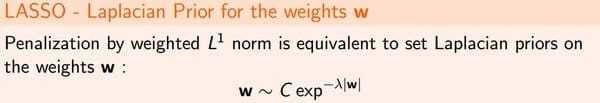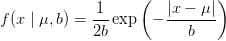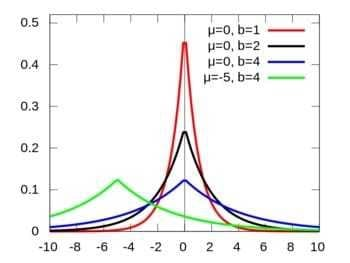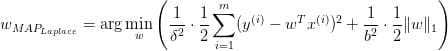### 3.4 Elastic Net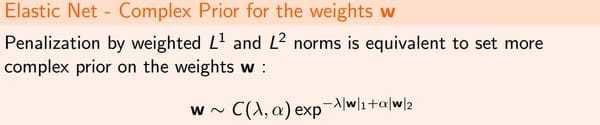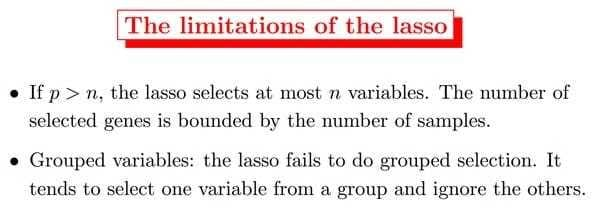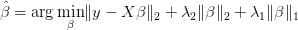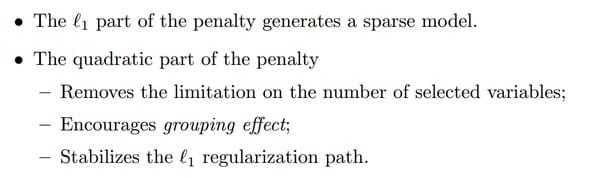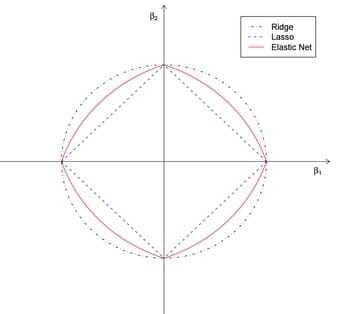### 3.5 总结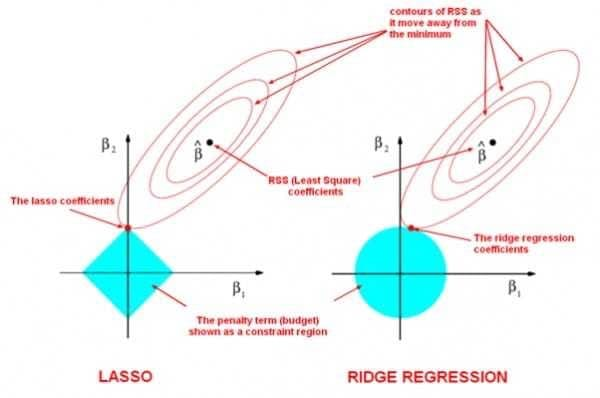Lazy Sparse Stochastic Gradient Descent for Regularized Mutlinomial Logistic Regression

-----------本文结束 感谢您的阅读-----------